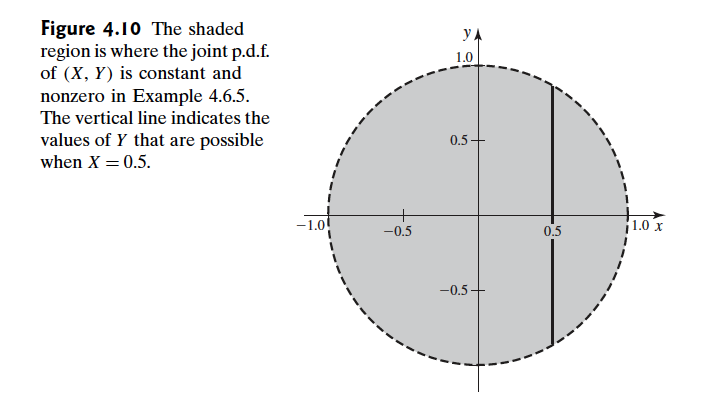Abstract: 本文介绍协方差和相关性的基础知识，以及部分性质
Keywords: Covariance,Correlation,Properties of Covariance and Correlation

# 协方差和相关性

## 协方差 Covariance

Definition Covariance. Let $X$ and $Y$ be random variables having finite means.Let $E(X)=\mu_X$ and $E(Y)=\mu_Y$ The covariance of X and Y,which is denoted by $Cov(X,Y)$ ,is defined as
$$Cov(X,Y)=E[(X-\mu_X)(Y-\mu_Y)]$$
if the expectation exists.

$$f(x,y)= \begin{cases} 2xy+0.5&\text{ for } 0\leq x\leq 1 \text{ and } 0\leq y\leq 1\\ 0&\text{otherwise} \end{cases}$$

\begin{aligned} \mu_X&=\int^{1}_{0}\int^{1}_{0}[2x^2y+0.5x]dydx\\ &=\int^{1}_{0}[x^2+0.5x]dx\\ &=\frac{7}{12} \end{aligned}

$$\int^{1}_{0}\int^{1}_{0}(x-\frac{7}{12})(y-\frac{7}{12})(2xy+0.5)dydx$$

Theorem For all random variables X and Y such that $\sigma^2_{X}<\infty$ and $\sigma^2_{Y}<\infty$ ,
$$Cov(X,Y)=E(XY)-E(X)E(Y)$$

\begin{aligned} Cov(X,Y)&=E(XY-\mu_X Y-\mu_Y X + \mu_X\mu_Y)\\ &=E(XY)-\mu_X E(Y)-\mu_y E(X) + \mu_X\mu_Y)\\ \end{aligned}

## 相关性 Correlation

Definition Correlation.Let X and Y be random variables with finite variances $\sigma^2_{X}$ and $\sigma^2_{Y}$ ,respectively. Then the correlation of $X$ and $Y$ ,which is denoted by $\rho(X,Y)$ ,is defined as follow:
$$\rho(X,Y)=\frac{Cov(X,Y)}{\sigma_X^2 \sigma_Y^2}$$

Theorem Schwarz Inequality.For all random variables $U$ and $V$ such that $E(UV)$ exists,
$$[E(UV)]^2\leq E(U^2)E(V^2)$$
If,in addition,the right-hand side of $[E(UV)]^2\leq E(U^2)E(V^2)$ is finite,then the two sides of it equal the same value if and only if there are nonzero constants $a$ and $b$ such that $aU+bV=0$ with probability 1.

1. 如果 $E(U^2)=0$ 那么 $Pr(U=0)=1$ 所以必然有 $Pr(UV=0)=1$ 那么 $E(UV)=0$ 于是不等式成立。
2. 同理可以证明 $E(V^2)=0$ 的情况。
3. 当 $E(U^2)$ 或者 $E(V^2)$ 为无限的时候，不等式也成立。
4. 接下来证明 $0 < E(U^2) < \infty$ , $0 < E(V^2) < \infty$ 的情况，对于所有的 $a$ 和 $b$ 那么：
不等式一：
$$0\leq E[(aU + bV)^2]=a^2E(U^2)+b^2E(V^2)+2abE(UV)$$
以及，不等式二：
$$0\leq E[(aU - bV)^2]=a^2E(U^2)+b^2E(V^2)-2abE(UV)$$
如果 令$a=[E(V^2)]^{1/2},b=[E(U^2)]^{1/2}$ 那么就有下面的关系：
不等式三：
$$E(UV)\geq -[E(U^2)E(V^2)]^{1/2}$$
根据不等式二，就有不等式四：
$$E(UV)\leq [E(U^2)E(V^2)]^{1/2}$$
上面两个不等式，不等式三和不等式四得出定理中的结论。
不等式中等号成立，当且仅当不等式三和不等式四等号成立，不等式三等号成立，当且仅当不等式一等于0成立，也就是当且仅当 $E[(aU+bV)^2]=0$ 成立，当且仅当 $aU+bV=0$ 恒成立。
同理可以得到 $aU-bV=0$ 恒成立，至此证毕！

Theorem Cauchy-Schwarz Inequality.Let $X$ and $Y$ be random variables with finite variance.Then
$$[Cov(X,Y)]^2\leq \sigma^2_X\sigma^2_Y$$
and
$$-1\leq \rho(X,Y)\leq 1$$
Furthermor,the inequality in $[Cov(X,Y)]^2\leq \sigma^2_X\sigma^2_Y$ is an equality if and only if there are nonzero constants $a$ and $b$ and a constant $c$ such that $aX+bY=c$ with probability 1.

Cauchy-Schwarz不等式，柯西是谁不介绍了，Schwarz翻译成中文叫施瓦茨。

1. 令$U=X-\mu_X$ 和 $V=Y-\mu_Y$
2. 根据协方差定理 $Cov(X,Y)=E(XY)-E(X)E(Y)$ 可以直接得到 $[Cov(X,Y)]^2\leq \sigma^2_X\sigma^2_Y$
3. 然后就可以得到 $-1\leq \rho(X,Y)\leq 1$ 这个结论

Definition Positively/Negatively Correlation/Uncorrelated.It is said that $X$ and $Y$ are positively correlated if $\rho (X,Y)>0$ ,that $X$ and $Y$ are negatively correlated if $\rho(X,Y) < 0$ ,and that $X$ and $Y$ are uncorrelated if $\rho(X,Y)=0$

## 相关性和协方差的的性质 Properties of Covariance and Correlation

If $X$ and $Y$ are independent random varibales with $0<\sigma^2_X<\infty$ and $0<\sigma^2_Y<\infty$ ,then
$$Cov(X,Y)=\rho(X,Y)=0$$

$$f(x)= \begin{cases} \frac{1}{2\pi}&\text{for } x^2+y^2 \leq 1\\ 0&\text{otherwise } \end{cases}$$Theorem Suppose that $X$ is a random variable such that $0<\sigma^2_X<\infty$ ,and $Y=aX+b$ for some constants $a$ and $b$ ,where $a\neq 0$ ,If $a > 0$ the $\rho(X < Y)=1$ If $a < 0$ ,then $\rho(X,Y)=-1$

1. 如果 $y=ax+b$
2. 那么 $\mu_Y=a\mu_X+b$ ,$Y-\mu_Y=a(X-\mu_X)$
3. 根据协方差定义有 $Cov(X,Y)=aE[(X-\mu_X)^2]=a\sigma^2_X$
4. 因为有 $\sigma_Y=|a|\sigma_X$ 所以定理结论得到证明 （这步可由柯西-施瓦茨不等式得出）
5. 证毕

Theorem If $X$ and $Y$ are random variables such that $Var(X)<\infty$ and $Var(Y)<\infty$ ,then
$$Var(X+Y)=Var(X)+Var(Y)-2Cov(X,Y)$$

\begin{aligned} Var(X+Y)&=E[(X+Y-\mu_X-\mu_Y)^2]\\ &=E[(X-\mu_X)^2+(Y-\mu_Y)^2+2(X-\mu_X)(Y-\mu_Y)]\\ &=Var(X)+Var(Y)+2Cov(X,Y) \end{aligned}

Corollary Let a,b and c be constants.Under the conditions of theorem upside
$$Var(aX+bY+c)=a^2Var(X)+b^2Var(Y)+2abCov(X,Y)$$

$$Var(X-Y)=Var(X)+Var(Y)-2Cov(X,Y)$$

Theorem If $X_1,\dots,X_n$ are random variables scuh that $Var(X_i)<\infty$ for $i=0,\dots,n$ then
$$Var(\sum^{n}_{i=1}X_i)=\sum^{n}_{i=1}Var(X_i)+2{\sum\sum}_{i<j}Cov(X_i,X_j)$$

1. 首先
$$Var(\sum^{n}_{i=1}X_i)=Cov(\sum^{n}_{i=1}X_i,\sum^{n}_{j=1}X_j)=\sum^{n}_{i=1}\sum^{n}_{j=1}Cov(X_i,X_j)$$
2. 把上面的求和分成两部分一部分是 $i=j$ 一部分是 $i\neq j$ ，因为 $Var(x_i,x_j)=Var(x_j,x_i)$
\begin{aligned} Var(\sum^{n}_{i=1}X_i)&=\sum^{n}_{i=1}Var（X_i）+{\sum\sum}_{i\neq j}Cov(X_i,X_j)\\ &=\sum^{n}_{i=1}Var(X_i)+2{\sum\sum}_{i<j}Cov(X_i,X_j) \end{aligned}

Corollary If $X_1,\dots,X_n$ are uncorrelated random varibales,then
$$Var(\sum^{n}_{i=1}X_i)=\sum^{n}_{i=1}Var(X_i)$$

0%